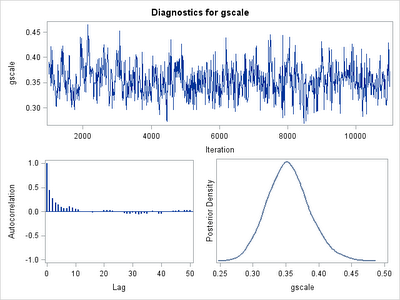Posts Tagged ‘ Bayesian methods ’

Example 9.8: New stuff in SAS 9.3– Bayesian random effects models in Proc MCMC

October 4, 2011
ByRounding off our reports on major new developments in SAS 9.3, today we'll talk about proc mcmc and the random statement.Stand-alone packages for fitting very general Bayesian models using Markov chain Monte Carlo (MCMC) methods have been available for...

Example 7.24: Sampling from a pathological distribution

March 1, 2010
ByEvans and Rosenthal consider ways to sample from a distribution with density given by:f(y) = c e^(-y^4)(1+|y|)^3where c is a normalizing constant and y is defined on the whole real line.Use of the probability integral transform (section 1.10.8) is not feasible in this setting, given the complexity of inverting the cumulative density function.The Metropolis--Hastings algorithm is a Markov...

Group-level variances and correlations

September 23, 2008
By

This is just a sum-up of what we should do when we try to estimate the group-level variances and correlations but the software failed to do so. As a R and lmer() user, I found this happens often.If this is the case, there are three potential problems...